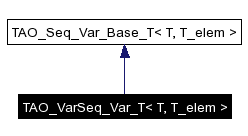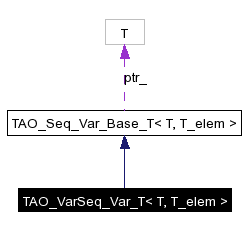# TAO_VarSeq_Var_T< T, T_elem > Class Template Reference

Parametrized implementation of _var class for sequences whose element is of variable size.. More...

`#include <Seq_Var_T.h>`

Inheritance diagram for TAO_VarSeq_Var_T< T, T_elem >:[legend]
Collaboration diagram for TAO_VarSeq_Var_T< T, T_elem >:[legend]
List of all members.

## Public Member Functions

TAO_VarSeq_Var_T (void)
TAO_VarSeq_Var_T (T *)
TAO_VarSeq_Var_T (const TAO_VarSeq_Var_T< T, T_elem > &)
TAO_VarSeq_Var_Toperator= (T *)
TAO_VarSeq_Var_Toperator= (const TAO_VarSeq_Var_T< T, T_elem > &)
T_elem & operator[] (CORBA::ULong index)
operator T *& ()

## Detailed Description

### template<typename T, typename T_elem> class TAO_VarSeq_Var_T< T, T_elem >

Parametrized implementation of _var class for sequences whose element is of variable size..

## Constructor & Destructor Documentation

 template ACE_INLINE TAO_VarSeq_Var_T< T, T_elem >::TAO_VarSeq_Var_T ( void )

 template ACE_INLINE TAO_VarSeq_Var_T< T, T_elem >::TAO_VarSeq_Var_T ( T * )

 template TAO_VarSeq_Var_T< T, T_elem >::TAO_VarSeq_Var_T ( const TAO_VarSeq_Var_T< T, T_elem > & )

## Member Function Documentation

 template ACE_INLINE TAO_VarSeq_Var_T< T, T_elem >::operator T *& ( )

 template TAO_VarSeq_Var_T< T, T_elem > & TAO_VarSeq_Var_T< T, T_elem >::operator= ( const TAO_VarSeq_Var_T< T, T_elem > & )

 template ACE_INLINE TAO_VarSeq_Var_T< T, T_elem > & TAO_VarSeq_Var_T< T, T_elem >::operator= ( T * )

 template ACE_INLINE T_elem & TAO_VarSeq_Var_T< T, T_elem >::operator[] ( CORBA::ULong index )

The documentation for this class was generated from the following files:
Generated on Sat Aug 6 03:19:37 2005 for TAO by1.3.9.1